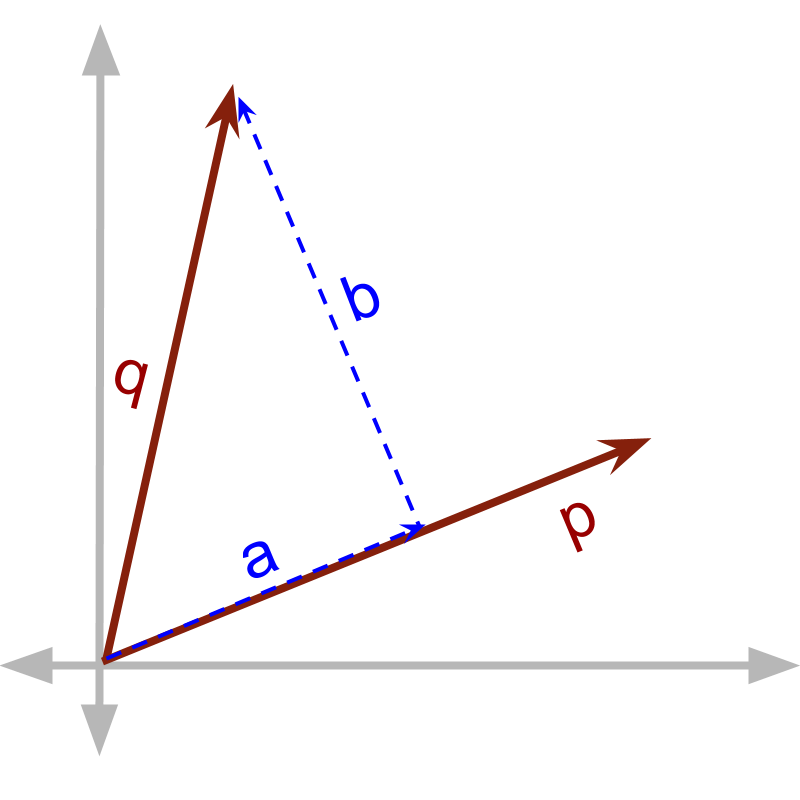maths > vector-algebra

Vector Arithmetic

what you'll learn...

Overview

Vector Arithmetic

»  Application Scenarios of Vector Arithmetic

→  Subtraction : inverse of addition

→  Repeated addition of a quantity: a vector is multiplied by a scalar

→  Product between two quantities : two vectors can be multiplied

»  Two Possibilities of Vector Multiplication→  Vector Dot Product : multiply with component in parallel

→  Vector Cross Product : multiply with component in perpendicular

»  Vector Division is not defined
→  Division is the inverse of multiplication. In vector multiplication, one of the components is lost in the product. And so vector division is not defined.

All these arithmetic operations are explained in detail later. The objective of this is to understand the role of components in parallel and in perpendicular.

recap

A person takes $2$$2$ apples and then takes $3$$3$ oranges. The person has total of $5$$5$ fruits. In this scalar quantities $2$$2$ and $3$$3$ add to the sum $5$$5$

A person takes $6$$6$ apples and then puts back $4$$4$ apples. After that, the person has '$2$$2$' apples. In this scalar quantity $4$$4$ is subtracted from $6$$6$ to get result $2$$2$.

Scalar quantities can be subtracted

A person takes $6$$6$ apples and then takes $-4$$- 4$ apples. Effectively the person has '$2$$2$' fruits. In this, $6$$6$ is added with $-4$$- 4$, which is effectively subtraction in the form $\left(6-4\right)$$\left(6 - 4\right)$.

Subtraction is the inverse of addition.

A person takes $2$$2$ apples and places them in his basket. He repeats that $3$$3$ times in total. Effectively, the person has $2+2+2=2×3$$2 + 2 + 2 = 2 \times 3$ apples. In this, scalar quantity $2$$2$ is repeatedly added $3$$3$ times to get result $6$$6$.

A scalar quantity can be added repeatedly.
Repeated addition is a form of multiplication.

A person having $10$$10$ apples in her basket, divides that equally to two kids. A kid will have $10÷2=5$$10 \div 2 = 5$ apples. In this, scalar quantity $10$$10$ is divided by $2$$2$.

A scalar quantity can be divided.

A person having $10$$10$ apples in her basket, gives half of them to two kids each. The kid has $10×\frac{1}{2}$$10 \times \frac{1}{2}$ or $10÷2$$10 \div 2$. In this $10$$10$ is multiplied by half, which is equivalently the division $10÷2$$10 \div 2$.

Division is the inverse of multiplication.

A person takes $3$$3$ oranges that costs $10$$10$ coins each. The person has to pay $3×10=30$$3 \times 10 = 30$ coins for the fruits. The scalar quantity $3$$3$ multiplies with another scalar quantity $10$$10$.

Two different scalar quantities can be multiplied.

Note: In this example, the number of oranges is forming a count equivalent to multiply the cost. Instead consider, force equals mass multiplied acceleration. In this, the mass and acceleration are multiplied. Neither of them is mapped to count equivalent.

Summary

•  Scalar quantities have magnitude measure.

•  Scalar quantities can be added ($2$$2$ fruit + $3$$3$ fruit $=5$$= 5$ fruit)

•  A scalar quantity can be multiplied (repeated addition) ($2$$2$ apples $3$$3$ times $=6$$= 6$ apples)

•  two or more scalar quantities can interact to form a product scalar quantity ($3$$3$ oranges at $10$$10$ coins each $=30$$= 30$ coins)

Apart from the addition and multiplication,

•  scalar quantities can be subtracted ($3$$3$ fruits $-2$$- 2$ fruits) $=1$$= 1$ fruit; which is inverse of addition

•  Scalar quantities can be divided, which is inverse of multiplication.

Fundamental mathematical operations

•  product of quantities (multiplication)

Let us see How these are defined for vectors that has magnitude and direction.

fundamental operations in vectors

A person walks $3$$3$ meter north and $4$$4$ meter east. The distance (in meter) from the starting point to the current position is '$\sqrt{{3}^{2}+{4}^{2}}$$\sqrt{{3}^{2} + {4}^{2}}$'. In this vector quantity $3$$3$ meter north and $4$$4$ meter east are added. We used coordinate geometry to figure out the result for now. We'll revisit for the definition of vector addition.

A person walks $3$$3$m in a direction and continues to do the same four times. The final position of him from the starting point is $3×4$$3 \times 4$ meter in the specified direction or $3+3+3+3$$3 + 3 + 3 + 3$ meter in the specified direction

Vector quantities can be repeatedly added or equivalently – vector can be multiplied by a scalar quantity.

A person pushes with 3 unit force toward east and causes 2 unit displacement towards north-east. Given that
work $=$$=$product of force and displacement
In this, 'force as a vector and displacement as a vector are multiplied'. Over this course, this will be explained in detail.

Vectors can be multiplied.

Vector Arithmetic involves

•  repeated addition or multiplication by scalar

•  two types of vector products (multiplication)

summary

Vector Arithmetic: Given vectors $\stackrel{\to }{p}$$\vec{p}$ and $\stackrel{\to }{q}$$\vec{q}$,

•  vector addition: $\stackrel{\to }{p}+\stackrel{\to }{q}$$\vec{p} + \vec{q}$

•  multiplication of vector by scalar: $\stackrel{\to }{p}+\stackrel{\to }{p}+\cdots \left(n-\text{times}\right)=n\stackrel{\to }{p}$$\vec{p} + \vec{p} + \cdots \left(n - \textrm{\times}\right) = n \vec{p}$

•  vector dot product: $\stackrel{\to }{p}$$\vec{p}$ multiplied by component of $\stackrel{\to }{q}$$\vec{q}$ in parallel to $\stackrel{\to }{p}$$\vec{p}$

•  vector cross product: $\stackrel{\to }{p}$$\vec{p}$ multiplied by component of $\stackrel{\to }{q}$$\vec{q}$ in perpendicular to $\stackrel{\to }{p}$$\vec{p}$

Apart from the addition, multiplication of vector by a scalar, and vector products,

•  vectors can be subtracted, which is 'inverse of addition' .

•  vectors can be divided by a scalar, which is included as 'inverse of multiplication of a vector by a scalar'

•  Vector division is not defined. We’ll see why in due course of this learning.

Outline